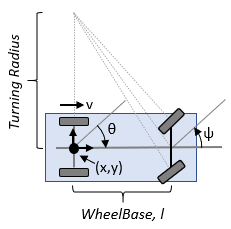# ackermannKinematics

Car-like steering vehicle model

## Description

`ackermannKinematics` creates a car-like vehicle model that uses Ackermann steering. This model represents a vehicle with two axles separated by the distance, WheelBase. The state of the vehicle is defined as a four-element vector, [x y theta psi], with a global xy-position, specified in meters. The vehicle heading, theta, and steering angle, psi are specified in radians. The vehicle heading is defined at the center of the rear axle. Angles are given in radians. To compute the time derivative states for the model, use the `derivative` function with input steering commands and the current robot state.## Creation

### Syntax

``kinematicModel = ackermannKinematics``
``kinematicModel = ackermannKinematics(Name,Value)``

### Description

example

````kinematicModel = ackermannKinematics` creates an Ackermann kinematic model object with default property values.```
````kinematicModel = ackermannKinematics(Name,Value)` sets additional properties to the specified values. You can specify multiple properties in any order.```

## Properties

expand all

The wheel base refers to the distance between the front and rear axles, specified in meters.

The vehicle speed range is a two-element vector that provides the minimum and maximum vehicle speeds, [MinSpeed MaxSpeed], specified in meters per second.

## Object Functions

 `derivative` Time derivative of vehicle state

## Examples

collapse all

Create a Robot

Define a robot and set the initial starting position and orientation.

```kinematicModel = ackermannKinematics; initialState = [0 0 0 0];```

Simulate Robot Motion

Set the timespan of the simulation to 1 s with 0.05 s timesteps and the input commands to 10 m/s and left turn. Simulate the motion of the robot by using the `ode45` solver on the `derivative` function.

```tspan = 0:0.05:1; cmds = [10 1]; % 10m/s velocity and left turn [t,y] = ode45(@(t,y)derivative(kinematicModel,y,cmds),tspan,initialState);```

Plot path

```figure plot(y(:,1),y(:,2))```Lynch, Kevin M., and Frank C. Park. Modern Robotics: Mechanics, Planning, and Control 1st ed. Cambridge, MA: Cambridge University Press, 2017.

##### Support평가판 신청